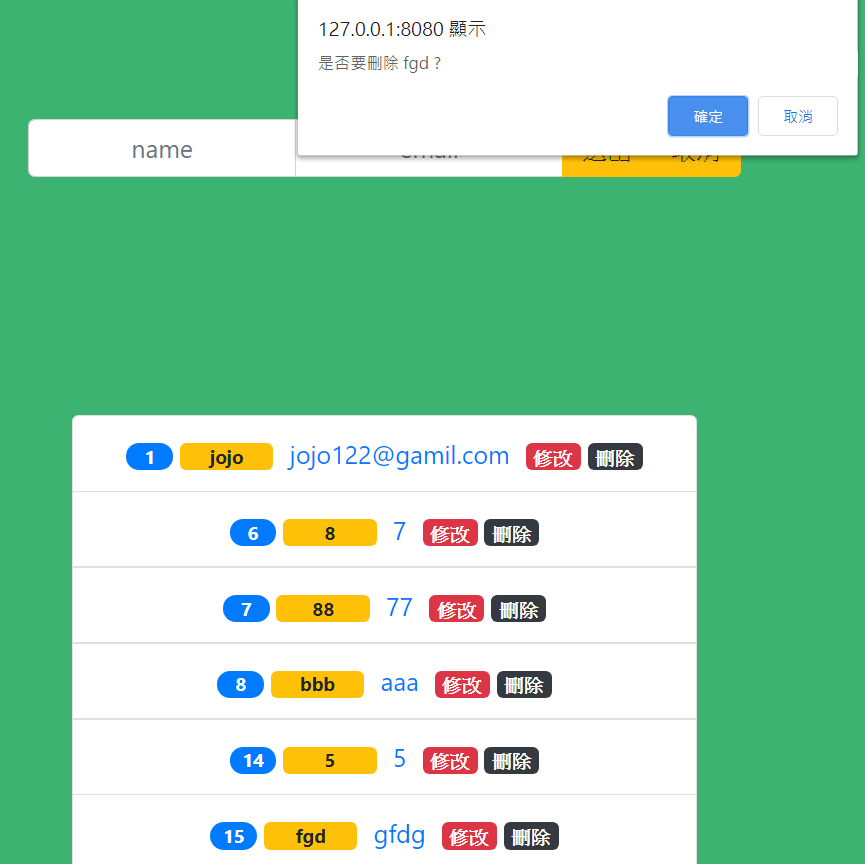#0

## Vue 畫面處理 & API 串接 自學筆記``````
<script src="https://cdnjs.cloudflare.com/ajax/libs/axios/0.18.0/axios.js"></script>

``````

``````            new Vue({
el: '#app',
data: {
// 保存聯絡人
contacts: [],
// 來判斷是否在修改
editIndex: null,
// 輸入的資料
input: {
name: '',
email: ''
}
},
``````

``````
</section>
<section v-else>
...

``````

``````
<div class="input-group">
<!-- .trim 去頭尾空白 -->
<input
type="text"
class="form-control"
placeholder="name"
v-model.trim="input.name"
/>
<input
type="text"
class="form-control"
placeholder="email"
v-model.trim="input.email"
/>
<div class="input-group-append">
<button
class="btn btn-warning"
type="button"
@click="submitHandler"
>
送出
</button>
<button
class="btn btn-warning"
type="button"
@click="cancelHandler"
>
取消
</button>
</div>
</div>

``````

``````
<li
class="list-group-item"
v-for="(item,index) in contacts"
>
</span>
{{item.name}}
</span>
<a
class="col-sm-4"
href="'mailto:'item.email"
>
{{item.email}}
</a>
<span
class="col-sm-1"
@click="editHandler(index)"
>修改</span>
<span
class="col-sm-1"
@click="delectHandler(index)"
>刪除</span>
</li>

``````

#### axios.get(url[, config])

``````
mounted() {
// 將路徑貼上
axios.get('http://localhost:8888/contcat')
.then((res) => {
console.log(res);
// 將獲取的資料同步到我們contacts
this.contacts = res.data;
})
.catch((err) => {
console.log(err);
})
},

``````

``````
cancelHandler() {
this.editIndex = null;
this.input.name = "";
this.input.email = "";
},

``````

#### axios.delete(url[, config])

``````
delectHandler(index) {
let target = this.contacts[index];
if (confirm(`是否要刪除 \${target.name} ?`)) {
// axios.delete(url[, config])
axios.delete('http://localhost:8888/contcat/' + target.id)
.then((res) => {
// 將input的資料push至畫面
// splice(index,1) 起始位置為傳入的下標，並刪除一個
this.contacts.splice(index, 1);
this.cancelHandler();

})
.catch((err) => {
console.log(err);
})
}

``````#### axios.post(url[, data[, config]])

``````
submitHandler() {
// 解構出資料name,email
let {
name,
email
} = this.input;
if (!name || !email) return
// 判斷是否為修改階段，如果不是才進去
if (this.editIndex == null) {
// 目標位址,資料,初始配置
// axios.post(url[, data[, config]])
// 將input送出的資料，同步更新到URL
axios.post('http://localhost:8888/contcat',
// this.input 也就是我們要更新上url的資料
// {name,email}
this.input
)
.then((res) => {
// 將獲取的資料(input的資料)push至畫面
this.contacts.push(res.data);
// 記得清空input
this.cancelHandler();
console.log(res.data, this.contacts);
})
.catch((err) => {
console.log(err);
})

``````

``````
editHandler(index) {
// 將點擊所要修改對應的name,email拆出來
let {
name,
email
} = this.contacts[index];
// 再將其要修改的值賦予至input
// this.input.name = name ;
// this.input.email = email ;

// 資料擺放順序並不會影響
this.input = {
email,
name
}
// 當在修改就賦值
this.editIndex = index;
}
},

``````

#### axios.put(url[, data[, config]])

``````
{
// 修改資料利用put一次將全部資料覆蓋
// axios.put(url[, data[, config]])
// url為當前要修改的那筆資料
axios.put('http://localhost:8888/contcat/' + this.contacts[this.editIndex].id,
this
.input)
.then((res) => {
console.log(this.input)
console.log(res.data);
// 將input的資料傳至畫面
this.contacts[this.editIndex] = res.data
this.cancelHandler();
})
.catch((err) => {
console.log(err);
})
}

``````

### 2 則留言

2

no027843 iT邦新手 5 級 ‧ 2020-07-23 18:07:05 檢舉

0